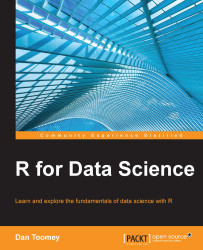•#### R for Data Science#### Overview of this book

R for Data ScienceCreditswww.PacktPub.comPrefaceFree Chapter
Data Mining PatternsData Mining SequencesText MiningData Analysis – Regression AnalysisData Analysis – CorrelationData Analysis – ClusteringData Visualization – R GraphicsData Visualization – PlottingData Visualization – 3DMachine Learning in ActionPredicting Events with Machine LearningSupervised and Unsupervised LearningIndex## Packages

In R, there are several packages that provide the correlation functionality to the programmer. We will be using the following packages in this chapter:

• `corrgram`: This is the tool to graphically display correlations

• `Hmisc`: This contains a variety of miscellaneous R functions

• `polycor`: This contains functions to compute polychoric correlations

• `ggm`: This contains functions for analyzing and fitting graphical Markov models

### Correlation

Basic correlation is performed in R using the `cor` function. The `cor` function is defined as follows:

```cor(x, y = NULL,
use = "everything",
method = c("pearson", "kendall", "spearman"))```

The various parameters of this function are described in the following table:

Parameter

Description

`x`

This is the dataset.

`y`

This is the dataset that is compatible with `x`.

`use`

This is the optional method for computing the covariance of missing values assigned. The choices are:

• `everything`

• `all.obs`

• `complete.obs`

• `na.or.complete`

• `pairwise.complete.obs`

`method...`